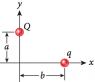# Maximizing x-component of force

• Physics2341313

#### Physics2341313

A charge Q is placed at a distance a = 3m and a charge q is placed at a distance b

For what value(s) of b is the x-component of the force maximized?

I know to maximize the force we need to maximize $F_Qq = k Qq b/(3^2 + b^2)^{3/2}$.

To do this we need to set the first derivative to zero and solve for b. Can someone walk me through this derivative? Having trouble with this and I can't seem to get it. The b in the numerator and 3/2 power came from taking the unit vector.

kQq are just constants so we just need to take the derivative of $b/(9 + b^2)^{3/2}$ no?

Need picture.

Here's the picture of the figure, where a = 3m in this specific case. I guess I should have also stated that the b in the numerator and the 3/2 power came from the cosine to be more correct in terminology?

#### Attachments

•P045figure.png
2.5 KB · Views: 325
x component of the force on which charge?
what are the signs of the charges? both +? both -? one each?

x-component on q, and the charge on each is positive.

x-component on q, and the charge on each is positive.
So if Q is restricted to the y axis, why wouldn't the answer be simply y=0?
The question would make more sense if it asked for the value of b to give the maximum y component on q.

I don't understand? Q is restricted on the y-axis at a height of a = 3m the charge q is arbitrary at a location b. The first part of the problem is to find the value b at which the x-component of the force between the two charges is at it's miniumum which would be at b = 0. To find it's maximum the problem at hand now the derivative is involved for its maximum value? Which is what I was having trouble with. I am not seeing how the y-axis comes into the problem since Q is fixed at that location and the location of q on the x-axis is variant.

NM, I read the problem backwards.
Your post 1 is OK. Why not finish the problem?
the y-axis comes into the problem since the x force on q is dependent on the value of a. But a is constant so proceed with d/db (Fx) = 0 etc.

Couldn't solve the derivative for b. I think I messed the derivative up I was getting high powers of b that couldn't be solved.

Sample of what I tried to solve...

$d/db [b/(9+b^2)^{3/2}] \Rightarrow [3b(9 + b^2)^{1/2} - (9 + b^2)^{3/2}]/(9 + b^2)^3$

After that it turned into a mess trying to solve for b

Ahh, nevermind I solved it. I forgot that I could discard the denominator when setting equal to zero. Made the problem more complex than need be,

Couldn't solve the derivative for b. I think I messed the derivative up I was getting high powers of b that couldn't be solved.

Sample of what I tried to solve...

$d/db [b/(9+b^2)^{3/2}] \Rightarrow [3b(9 + b^2)^{1/2} - (9 + b^2)^{3/2}]/(9 + b^2)^3$

After that it turned into a mess trying to solve for b
First thing is you throw out the denominator (why can you do that?)
secod thing is you have the sign of the numerator wrong d(uv) = (v du - u dv)/v2.
third thing is you didn't do the derivative right. One way to check is again using dimensions. Ignoring the denominator, your 1st term on the right has dimension L2 while the second has L3 where L = length.
If you divide the numerator by a certain quantity the solution becomes very easy.
finally a small point: don't write "9", leave as a2 until the end. Too many numbers mess up the math and make checking harder.
gotta go to bed (where are you located? in Hawaii or U.K.?Ahh, nevermind I solved it. I forgot that I could discard the denominator when setting equal to zero. Made the problem more complex than need be,
OK. Good! Should still look at my post #11 for one or two points.